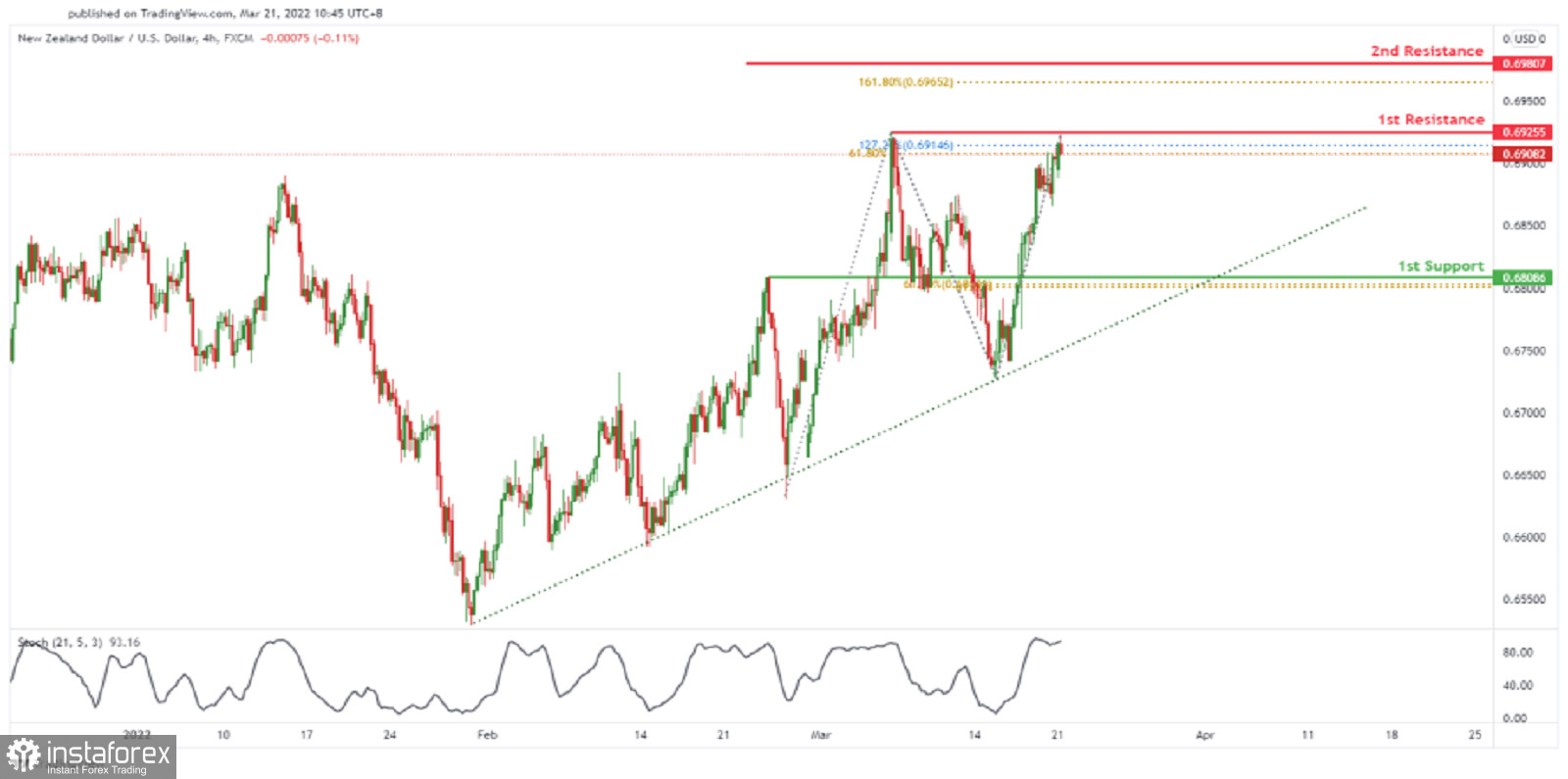# FX.co ★ NZDUSD Potential For Bearish Reversal | 21th March

Forex Analysis:::2022-03-21T06:15:08

## NZDUSD Potential For Bearish Reversal | 21th MarchOn the H4, price is expected to drop closer to the ascending trendline. Price is near 1st resistance level of 0.69255 in line with 61.8% Fibonacci retracement and 127.2% Fibonacci extension. Price can move towards the 1st support level of 0.68086 which is in line with 61.8% Fibonacci retracement and 61.8% Fibonacci projection. Our bearish bias is supported by the stochastic indicator where it is at resistance level. Alternatively, price might go up to the 2nd resistance level at 0.69807 which is in line with 161.8% Fibonacci extension.

Entry: 0.69255

Reason for Entry: 61.8% Fibonacci retracement and 127.2% Fibonacci extension.

Take Profit: : 0.68086

Reason for Take Profit: 61.8% Fibonacci retracement and 61.8% Fibonacci projection.

Stop Loss: 0.69807

Reason for Stop Loss: 161.8% Fibonacci extension.

Analyst InstaForex